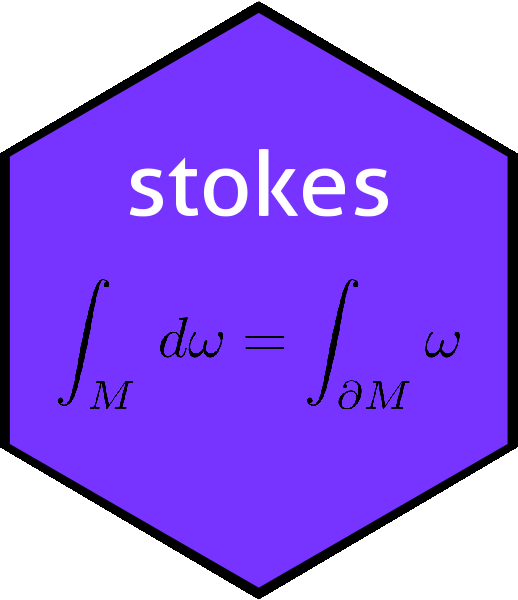# The hodge() function in the stokes packagehodge
## function (K, n = dovs(K), g, lose = TRUE)
## {
##     if (missing(g)) {
##         g <- rep(1, n)
##     }
##     if (is.empty(K)) {
##         if (missing(n)) {
##             stop("'K' is zero but no value of 'n' is supplied")
##         }
##         else {
##             return(kform(spray(matrix(1, 0, n - arity(K)), 1)))
##         }
##     }
##     else if (is.volume(K, n)) {
##         return(scalar(coeffs(K), lose = lose))
##     }
##     else if (is.scalar(K)) {
##         if (missing(n)) {
##             stop("'K' is scalar but no value of 'n' is supplied")
##         }
##         else {
##             return(volume(n) * coeffs(K))
##         }
##     }
##     stopifnot(n >= dovs(K))
##     f1 <- function(o) {
##         seq_len(n)[!seq_len(n) %in% o]
##     }
##     f2 <- function(x) {
##         permutations::sgn(permutations::as.word(x))
##     }
##     f3 <- function(v) {
##         prod(g[v])
##     }
##     iK <- index(K)
##     jj <- apply(iK, 1, f1)
##     if (is.matrix(jj)) {
##         newindex <- t(jj)
##     }
##     else {
##         newindex <- as.matrix(jj)
##     }
##     x_coeffs <- elements(coeffs(K))
##     x_metric <- apply(iK, 1, f3)
##     x_sign <- apply(cbind(iK, newindex), 1, f2)
##     as.kform(newindex, x_metric * x_coeffs * x_sign)
## }
## <bytecode: 0x55abbc2a2438>
## <environment: namespace:stokes>

Given a $$k$$-form $$\omega$$, function hodge() returns its Hodge dual $$*\omega$$ or $$\star\omega$$. Formally, if $$V={\mathbb R}^n$$, and $$\Lambda^k(V)$$ is the space of alternating linear maps from $$V^k$$ to $${\mathbb R}$$, then $$\star\colon\Lambda^k(V)\longrightarrow\Lambda^{n-k}(V)$$.

To define the Hodge dual, we need an inner product $$\left\langle\cdot,\cdot\right\rangle$$ [function kinner() in the package] and, given this and $$\beta\in\Lambda^k(V)$$ we define $$\star\beta$$ to be the (unique) $$n-k$$-form satisfying the fundamental relation:

$\alpha\wedge\left(\star\beta\right)=\left\langle\alpha,\beta\right\rangle\omega,$

for every $$\alpha\in\Lambda^k(V)$$. Here $$\omega=e_1\wedge e_2\wedge\cdots\wedge e_n$$ is the unit $$n$$-vector of $$\Lambda^n(V)$$. Taking determinants of this relation shows the following.

If we use multi-index notation so $$e_I=e_{i_1}\wedge\cdots\wedge e_{i_k}$$ with $$I=\left\lbrace i_1,\cdots,i_k\right\rbrace$$, then

$\star e_I=(-1)^{\sigma(I)}e_J$

where $$J=\left\lbrace j_i,\ldots,j_k\right\rbrace=[n]\backslash\left\lbrace i_1,\ldots,i_k\right\rbrace$$ is the complement of $$I$$, and $$(-1)^{\sigma(I)}$$ is the sign of the permutation $$\sigma(I)=i_1\cdots i_kj_1\cdots j_{n-k}$$. We extend to the whole of $$\Lambda^k(V)$$ using linearity.

Package idiom is straightforward:

(o <- rform())
## An alternating linear map from V^3 to R with V=R^7:
##  -8 dNA^dNA^dNA +6 dx^dNA^dNA -5 dy^dNA^dNA -4 dx^dz^dNA +3 dy^dz^dNA +9 dx^dy^dNA -7 dx^dNA^dNA -2 dy^dz^dNA + dx^dNA^dNA
hodge(o)
## An alternating linear map from V^4 to R with V=R^7:
##  + dy^dz^dNA^dNA -2 dx^dNA^dNA^dNA -7 dy^dz^dNA^dNA +9 dz^dNA^dNA^dNA -3 dx^dNA^dNA^dNA +4 dy^dNA^dNA^dNA +5 dx^dz^dNA^dNA -6 dy^dz^dNA^dNA -8 dx^dy^dz^dNA

We verify that the fundamental relation holds by direct inspection:

o ^ hodge(o)
## An alternating linear map from V^7 to R with V=R^7:
##  +285 dx^dy^dz^dNA^dNA^dNA^dNA
kinner(o,o)*volume(dovs(o))
## An alternating linear map from V^7 to R with V=R^7:
##  +285 dx^dy^dz^dNA^dNA^dNA^dNA

showing agreement. We may work more formally by defining a function that returns TRUE if the left and right hand sides match

diff <- function(a,b){a^hodge(b) ==  kinner(a,b)*volume(dovs(a))}

and call it with random $$k$$-forms:

diff(rform(),rform())
##  TRUE

Or even

all(replicate(10,diff(rform(),rform())))
##  TRUE

## Small-dimensional vector spaces

We can work in three dimensions in which case we have three linearly independent $$1$$-forms: $$dx$$, $$dy$$, and $$dz$$. To work in this system it is better to use dx print method:

options(kform_symbolic_print = "dx")
hodge(dx,3)
## An alternating linear map from V^2 to R with V=R^3:
##  + dy^dz

# Non positive-definite metrics

The inner product $$\left\langle\alpha,\beta\right\rangle$$ above may be generalized by defining it on decomposable vectors $$\alpha=\alpha_1\wedge\cdots\wedge\alpha_k$$ and $$\beta=\beta_1\wedge\cdots\wedge\beta_k$$ as

$\left\langle\alpha,\beta\right\rangle= \det\left(\left\langle\alpha_i,\beta_j\right\rangle_{i,j}\right)$

where $$\left\langle\alpha_i,\beta_j\right\rangle=\pm\delta_ij$$ is an inner product on $$\Lambda^1(V)$$ [the inner product is given by kinner()]. The resulting Hodge star operator is implemented in the package and one can specify the metric. For example, if we consider the Minkowski metric this would be $$-1,1,1,1$$.

To work with $$2$$-forms in relativistic physics, it is often preferable to use bespoke print method usetxyz:

o <- kform_general(4,2,1:6)
o
## An alternating linear map from V^2 to R with V=R^4:
##  +6 dz^dNA +5 dy^dNA +4 dx^dNA +3 dy^dz +2 dx^dz + dx^dy
options(kform_symbolic_print = "txyz")
o
## An alternating linear map from V^2 to R with V=R^4:
##  +6 dy^dz +5 dx^dz +4 dt^dz +3 dx^dy +2 dt^dy + dt^dx

Function hodge() takes a g argument to specify the metric:

hodge(o)
## An alternating linear map from V^2 to R with V=R^4:
##  + dy^dz -2 dx^dz +3 dt^dz +4 dx^dy -5 dt^dy +6 dt^dx
hodge(o,g=c(-1,1,1,1))
## An alternating linear map from V^2 to R with V=R^4:
##  - dy^dz +2 dx^dz +3 dt^dz -4 dx^dy -5 dt^dy +6 dt^dx
hodge(o)-hodge(o,g=c(-1,1,1,1))
## An alternating linear map from V^2 to R with V=R^4:
##  +8 dx^dy -4 dx^dz +2 dy^dz